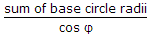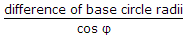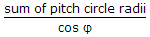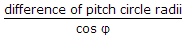# Mechanical Engineering - Theory of machines - Discussion

22.

The centre distance between two meshing involute gears is equal to

 [A].[B].[C].[D].Explanation:

No answer description available for this question.

 Roopkanwar said: (Nov 16, 2015) It's a formula that is equal to C1+C2/cos (phi). Where C1 and C2 are base circle diameter.

 Dayanand Yadav said: (Jul 5, 2016) @Roopkanwar. C1 and C2 are base circle radii, not the diameter.

 Nabam said: (Sep 21, 2016) It's more like base circle diameter equal to pitch circle diameter multiplied by cos (φ).